# Operation Sense, Tool-Based Pedagogy, Curricular Breadth: A Proposal

by Henri Picciotto

This is a chapter from a book that apparently never came out: Employing Children's Natural Powers to Build Algebraic Reasoning in the Context of Elementary Mathematics (James Kaput, editor).

I wrote it in 1995, and made a few revisions when I posted it on this Web site in 1998.

Article outline:
Making Sense
Operation Sense
Sample Lessons
A Conceptual Approach
The Distributive Law
Manipulatives
Base Ten Blocks and the Lab Gear
Polemics
Tool-Based Learning
Fun Math
Conclusion
Bibliography

The reform movement has promoted a shift in the central goal of school mathematics: from the memorization of algorithms to conceptual understanding. Number sense and function sense have been widely seen as key components of this understanding. In this chapter, I propose that:

• Operation sense is the key link between number sense, function sense, and the all-important symbol sense. Operation sense, therefore, deserves to be at the heart of early mathematics.
• A good way to support the shift towards understanding is with a tool-based pedagogy: the use of manipulative, pictorial, and electronic thinking tools. The use of such tools does not guarantee understanding, but it does provide a good environment for discussion, communication, and reflection, and can play an important role in rethinking early mathematics, including the necessary changes in teacher education.
• The (enormously important) domain of arithmetic should not be the whole of elementary school mathematics. An eclectic collection of topics drawn from recreational math and elsewhere can provide a rich source of contexts for worthwhile early explorations.
• The implementation of this or any significant reform of elementary school mathematics will require a political strategy. The centerpiece of such a strategy should be the training and hiring of math specialists throughout our elementary schools.

## Making Sense

There is broad agreement in the math reform movement that schools' emphasis on algorithmic mastery in both arithmetic and algebra is misguided. The students trained under this regime fail to develop an understanding of the underlying mathematics, and in fact soon lose their grasp on the very skills that were intended to be the focus of their education. To address this problem, the reform movement proposes changes in direction and emphasis, in both curriculum and pedagogy. These changes are often presented as a way to help students develop number sense, and function sense.

The "sense" formulation is an improvement in our view of students and learning. It pushes us to a view of the student as a thinker, a person capable of understanding important mathematical domains, and helps move our thinking away from the view of the student as a programmable machine, a view that is consistent with the "skills" emphasis, but inconsistent with the reality of young human beings.

In the domain of arithmetic, instead of spending hundreds of hours trying to make children into a poor substitute for a 5 dollar machine, we should shift to the development of number sense, mental arithmetic, and an introduction to number theory. While much can be learned by discussing and thinking about ways to carry out calculations, accuracy and speed are no longer the goal: understanding is. A similar shift is necessary in algebra. In "A New Algebra" (Picciotto and Wah, 1993) I articulated a proposal for new directions in secondary school algebra. Not surprisingly, a necessary corollary to taking these new directions is to make some changes at the elementary level.

A key tenet of the new algebra I propose is the emphasis on the development of symbol sense. A recent paper by Abraham Arcavi (1994) helps focus our attention on this. I fear symbol sense may be undervalued by some in the reform movement. In some cases, an overreaction to the failure of our current approach to Algebra may lead to a badmouthing of symbolic representation and manipulation. Such an over-reaction would only serve to perpetuate the current situation, but in a different way. We would go from algebra as gatekeeper through ineffective teaching of symbol sense (College Entrance Examination Board, 1990) to lack-of-algebra as gatekeeper through out-and-out non-teaching of symbol sense.

Many beginners in algebra confuse 2x, 2+x, and x2. This is not merely a linguistic obstacle, but also a conceptual and mathematical one. The notation would be easier to grasp if it were associated with some meaning in the minds of the students. Another popular mistake among high school students is to "distribute the square": (x+5)2 = x2 + 25. It is easy to blame this on the weaknesses of Algebra 1, but some questions remain: Do we think it is important for students to understand this? If yes, how would one go about getting such ideas across?

If students cannot perform simple algebraic manipulations correctly, they cannot effectively pursue math, science, or statistics. I am not talking about simplifying radicals within fractions within radicals within fractions, but simple manipulations like the one above, or like removing the parentheses in -(y-1). The reality is that even simple manipulations are difficult to understand for many, if not most students. That difficulty leads many educators to despair about the possibility of teaching them, and makes it tempting to conclude that these manipulations are not important. On the other hand, the fact that these are seen as trivial by many of us leads us to hope (foolishly) that they will take care of themselves if we only engage the students in interesting math problems.

Students who cannot work with symbols are severely handicapped. This is true whether their lack of facility with symbols stems from being victims of the old-fashioned Algebra 1, or participants in a reform curriculum that de-emphasizes symbolism to the point of near-omission. Just like number sense has not and cannot be made obsolete by calculators, symbol sense is a necessary part of math and science, and cannot be made obsolete by technology. School algebra is not the only conceivable symbolic framework, (computer programming offers an interesting alternative), but it is a very important one. In fact the computer programs beneath any new computer-based symbolic representations themselves depend on the use of traditional algebraic notation.

## Operation Sense

Conceptually, number sense, function sense, and symbol sense involve a substantial common component which I would like to call operation sense. For example, take the sequence 5, 8, 11, 14, ...

• Number sense should include the ability to recognize repeated addition in this sequence, and its relationship to multiplication.
• Symbol sense should include the ability to express and recognize the same thing in a form such as a+nd, or in this case 5+3n.
• Function sense should include the ability to recognize the relationship between that and the general linear function y=mx+b -- here y=3x+5.

None of this is possible without a solid grasp of addition and multiplication, their structural relationship, and their uses in various applications. An understanding of operations is the foundation of number sense, symbol sense, and function sense.

While it may be interesting to some to distinguish algebraic from arithmetic thinking, it is useful to recognize operations as the joint underlying foundation of both arithmetic and algebra (whether you see algebra as being about functions, about structure, or like I do see it as a combination of those). This has immediate curriculum consequences, irrespective of the eventual resolution of the broader theoretical questions.

## Sample Lessons on Operations

When I taught elementary school, I taught many lessons to help my students develop operation sense. Many of these lessons appear, in a secondary school version, in Algebra: Themes, Tools, Concepts (a secondary textbook I helped write -- Wah and Picciotto, 1994).

### The Zero Monster:

The Zero Monster eats zeros, but there are no zeros in sight. However, there are some cups and some caps [figure 1]. Cups and caps cannot be turned over, but a cup can be combined with a cap to make a zero, which the Monster can eat. For example, if there are seven cups and three caps, you can make three zeros. After the Monster eats, there are four cups left. So you can say that 7 cups + 3 caps = 4 cups.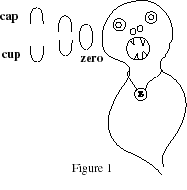This setup leads to various addition problems (all cups, all caps, cups plus caps, caps plus cups, not to mention missing addend problems of the various types. An interesting problem is "what Zero Monster additions have answer 4 cups?" ) Zero Monster arithmetic is of course isomorphic to the integers under addition.

This lesson is meant to complement, not replace other approaches to integer arithmetic, such as the particle / antiparticle model, the elevator model, the put in / take out model, the 1-D vector model, the income / expense model, and the motions on the number lines model. The question is not "which is the best model?" but "how do we use and articulate multiple models when teaching important concepts?"

### The McNuggets Problem

At McDonald's you can order 6, 9 or 20 Chicken McNuggets. This allows you to order 15 McNuggets (6+9) but not 7 McNuggets. What numbers can be ordered? What numbers cannot be ordered?

This problem, with a number theoretic flavor, is an example of what I believe is called the "stamp problem". (As in: What values can you get by combining any number of 23 cent and 32 cent stamps?) In the case of two stamps with relatively prime values, there is a nice result about the greatest value that cannot be obtained. However, at the elementary school level, that result is not as important as the experience with addition, multiplication, and their relationship that students gain while exploring it.

Another rich problem from the same domain that works well in elementary school: In how many ways can each whole number (or integer) be written as a sum of consecutive whole numbers (or integers)? Again, the full answer need not be arrived at in order to get a good mental workout and develop an understanding about numbers and operations.

### Mod Clocks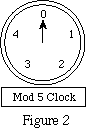A mod clock [figure 2] is a talking function machine. If you say a whole number, it responds with a whole number. For example, one mod clock responded this way:

7 --> 2
11 --> 1
4 --> 4
17 --> 2
10 --> 0

Students try numbers as inputs, and the clock responds (in the voice of the teacher) with a number from 0 to 4. The output is the remainder when dividing the student's number by 5, but at first students do not see this. They are able to predict the output based on discovering a pattern relating the last digit of the input to the output. However when asked what the special number of this clock is, it is not unusual for a student to say "5". Matters are clarified further by analyzing the behavior of other mod clocks, such as (in rough order of difficulty) mod 2, mod 9, mod 3, mod 4, mod 6.

At some point in this process, it becomes useful to discuss strategies for guessing the output. In some cases you can inspect the number's last digit, in other cases the sum of the digits is useful. In all cases, it is possible to count around the clock, and see where one lands -- except of course for very large numbers, where such a strategy is not likely to succeed.

Some mod clocks ding audibly before giving their outputs. For example, in the case of the mod 5 clock:

7 --> ding, 2
11 --> ding, ding, 1
4 --> 4
17 --> ding, ding, ding, 2
10 --> ding, ding, 0

The number of dings corresponds to the number of times one passes 0 when counting to the input number, or in other words the quotient of the division by 5. Again, students can be asked to predict the number of dings, and worthwhile discussions can be had about how this all works.

It would not be difficult to create a Mod Clock computer microworld, but the activity works well without a computer.

This work can be followed up by defining addition and multiplication mod 5 (or 9, etc). Students can be asked to look for the identity element, the inverse of each element if it exists. They can make addition and multiplication tables, solve missing addend problems, and so on. Mod clocks make for explicit discussion of operations, and for deeper understanding of the structures underlying the real number system.

A related area to investigate is Calendar Math, where the current month is declared to be infinite, and an arithmetic of the days of the week is created. For example, assuming the month starts on a Wednesday, the sum We+Th --> 1+2=3 --> Fr. Interestingly, you would get We+Th=Fr even if you picked different dates for Wednesday and Thursday, such as 8+9=17, or 1+9=10. The 3rd, the 10th and the 17th are all Fridays. (Of course, if the month did not start on Wednesday, everything would be different.) An early question in the investigation of Calendar Math is what day of the week would the 100th day of the month be? (The infinite month is needed in order to make it possible to use late dates in additions or multiplications without falling out of the month.) Again, one can define multiplication, make tables, solve missing addend and factor problems, find the identity and inverse elements, in short explore the properties of these operations.

### Magic Carpets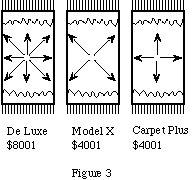Magic carpets are the only means of transportation available to travel among the lattice points. They come in various configurations [Figure 3]. A carpet can be equipped with one to eight arrows: East, West, North, South, North-East, North-West, South-East, and South-West. For example, a carpet equipped with a North arrow, a North-East arrow, and a South arrow makes it possible to go from the origin to (3,2) in many ways. Two examples:

NE, NE, NE, S -- which could be abbreviated to (NE)3*S
S, NE, S, NE, S, NE, N, N -- which could be abbreviated to (SNE)3*N2

Many questions can be investigated in this microworld, involving notation, concepts, and the interaction of the two. For example: what sequences of moves take you back where you started? what sequences of moves are equivalent to each other? for a given destination, what is the most economical way to get there? what are the rules for simplifying sequences of moves? what are the "fastest" carpets? what are the carpets that can get most places with the fewest arrows? what is the greatest number of arrows a carpet may have and still not be able to reach every lattice point?

### The YZ game

The goal is to shorten strings of Ys and Zs, using the following rules:

YZ = ZY
YYY can be erased
ZZ can be erased
For example: YZYZZYZZZYY
erase the first ZZ: YZYYZZZYY
erase the first ZZ: YZYYZYY
switch the first YZ: ZYYYZYY
erase YYY: ZZYY
erase ZZ: YY
YY cannot be simplified

After practicing by shortening YZ strings on the chalkboard, students can be asked to predict the simplified version of a given string. What are the possible resulting strings? How should we write the empty string? What is the inverse of a given string? A "put together table" for the six elements of the group can be filled in. Exponential notation can be introduced, and strings like Y9 Z9 can be discussed.

A follow-up is "the yz game", where the rules are:

z = yzy
yyy can be erased
zz can be erased

No commutativity this time. This is very un-intuitive, compared to the previous example. Predictions of the simplified version of a given string are extremely difficult to make, and strategies for simplifying a string as simple as zyz are worth discussing.

Another follow-up: the "def" game (presented in story form in Wah and Picciotto, 1994, p. 112). In this game, there are three symbols rather than two, and the rules are:

dd=e
ee=f
ff=d

How many distinct elements are there? What is the identity element? what is the inverse of each element? What are the powers of each element? The fact that this group turns out to be isomorphic to the (additive) calendar group discussed above is beyond elementary school students' understanding, but may perhaps be discussed profitably with their teachers.

## A Conceptual Approach to Operations

The main purpose of the sample lessons presented above is to lead students to pay explicit attention to operations and to their structure. Most of them have a strong group theoretic flavor. Surprisingly, (or perhaps not!), I have found that elementary school students are far more open to these sorts of explorations than high school students, who by then reflect the dominant culture and fear that such work may be frivolous and lack "real world" applicability. Of course, the lessons above only scratch the surface. Much more work on operations needs to be done in elementary school, for example on:

• undoing operations,
• place value,
• powers of ten,
• order of operations,
• rational numbers,
• the effect of multiplying and dividing by numbers between zero and one,

and so on.

By the time students reach secondary school, they should be acquainted with the following ideas:

1. Models, interpretations, and uses of the four operations. (In middle school include: squares and square roots; cubes; an introduction to exponentiation.) In particular, this entails the ability to recognize which operation can be used, and how, to solve various problems, as well as the ability to recognize whether one divides one number by the other, or vice-versa.
2. The structural relationships between operations, especially the inverse relationship between addition and subtraction, and multiplication and division, and also the distributive law. (In middle school, include an introduction to the laws of exponents.)
3. Generalization of the concept of operation beyond arithmetic by looking at concrete examples of groups. Familiarity with the concept of identity and inverse element, commutativity and non-commutativity. (This point is not intended to promote rote parroting of formalistic rules. I hope the sample lessons showed that these ideas can be approached at an intuitive and informal level.)

In addition to lessons such as the ones mentioned above, the work described in just about every chapter of this volume can help develop these understandings, provided that the appropriate awareness is present among the teachers. The purpose of the conceptual and structural view of the operations is not so much to prepare students for college-level abstract algebra, a goal that is only relevant to a tiny fraction to our students. Rather, the purpose is to strengthen our students' grasp of number, function and symbol, which in turn will help prepare them for any sort of work in mathematics and science, including especially algebra -- however you define it.

Speed and accuracy in executing traditional algorithms for carrying out the operations should not be the goal of instruction. Instead, discussion and analysis of student-generated and traditional algorithms should have the goal to improve students' conceptual understanding of the operations and of the number system. Such discussion of division algorithms can for example reveal the structural relationship between division and multiplication, and also between division and repeated subtraction or addition.

Separating long division as a skill (not a very useful one these days) from the concepts related to division is representative of the approach I propose. The concepts will never be obsolete. A high school parallel may help make the point: I do not teach my high school students how to use a slide rule accurately and fast, since that skill is obsolete. But I try to teach them why and how a slide rule works, because this allows me to get to key conceptual points about logarithms. The same approach applies to traditional arithmetic algorithms. Being able to deconstruct them is worthwhile. Being able to use them accurately and fast, less so.

The conceptual emphasis does not mean that we can ignore "grammatical" issues, such as how to read expressions that involve minus, or expressions that involve several operations. When working on problems beyond a certain level of complexity, expressions such as -(1 + (-2) - 3) will surface. This expression includes three minus signs, each of which has a different interpretation. The last one, between two sub-expressions, means "subtract," or "take away." The first one, in front of an expression, means "the opposite of". The middle one, in front of a positive number, means "negative". These notational issues cannot and should not be avoided. Of course, to a great extent, they are questions of convention and not of principle, and should be treated as such, but that does not mean they are not important.

Another example of a grammatical nature: students should be able to tell that an expression like 2(3) + 1 is an addition, not a multiplication, because the + is about the whole expression, while the implied multiplication sign only affects the 2 and the 3. On the other hand, the expression 2(3+1) is a multiplication, because the implied multiplication sign is about the whole expression, while the + only involves the 3 and the 1. Again, this is a question of convention, but it is related to an important structural concept: the distributive law.

## The Distributive Law

The most crucially important and difficult structural understanding about operations at the elementary level is the distributive law. Understanding it is fundamental to doing mental arithmetic, as in: 24 * 30 = 600 + 120. This sort of mental agility with numbers remains important in an age when electronic computation increasingly replaces paper-pencil digit-pushing. (Of course there are other important understandings that go into number sense, such as especially place value.)

The distributive law is also a key concept in the development of algebraic symbol sense. Abraham Arcavi (1994) attempts to define symbol sense by discussing a number of examples, several of which are built around the distributive law. For example, understanding the distributive law allows one to see that the equation (2x+3)/(4x+6)=2 does not have any solution. Or take the problem: "Take an odd number, square it, and then subtract 1. What can be said about the resulting number?" Writing the expression (2n-1)2-1, and using the distributive law repeatedly, we get:

(2n-1)2-1 = 4n2-4n = 4n(n-1) = 8*n(n-1)/2

This allows the insight that the resulting numbers are triangular numbers multiplied by 8. Arcavi's discussion of this example does not focus on the distributive law, but on the ability of an expert to read into the symbols: the second expression tells us that the number is a multiple of 4, the third that since n and n-1 are consecutive, it must be an even multiple of 4, ie a multiple of 8, and finally the last expression that it is a triangular number times 8. However the example also shows that it is difficult to separate interpretive ability from manipulative facility. The insights the symbols give us can only be there for us if we understand the underlying structure.

There has been a fair amount of bashing of factoring in the math reform movement. This probably stems from the overemphasis that many teachers and textbooks have put on the skill of factoring trinomials at the Algebra 1 level. However, if students do not understand the concept of factoring, they do not fully understand multiplication or the distributive law, and they are essentially illiterate in the symbolic realm. Once again, I suggest we make a distinction between skill and concept. Factoring, as a skill, should be de-emphasized. Factoring, as a concept, should be given more emphasis. It is a particular case of reversibility, "an ability to restructure the direction of a mental process from a direct to a reverse train of thought" (Krutetskii, quoted by Rachlin, whose perceptive paper "Algebra from x to why" (1987) should be required reading for all who profess an interest in the learning of algebra).

## Manipulatives

"Geometry is the user interface for mathematics." (Attributed to Bill Thurston.)

It is widely believed that working with numbers automatically generates an understanding of such concepts as the distributive law. Many teachers and textbooks describe algebra as the "natural" extension and generalization of arithmetic. And of course, there is some validity to that point of view. However the fact of the matter is that many students have tremendous difficulty making the transition to these ideas with variables, perhaps because they have a shaky sense of number. In any case, work with well-designed manipulatives can help build the necessary foundation to facilitate the leaps to abstraction that are embedded and embodied in the notation of algebra. For some students manipulatives provide an important tool, for others, they provide a geometric context where they can broaden and deepen their understanding, which is often only mechanical mastery of algorithms.

Over the years Zoltan Dienes, Mary Laycock, and Peter Rasmussen each contributed some useful ideas to the design of effective algebra manipulatives. I took their work further and developed the Lab Gear, a manipulative that has helped many teachers bring symbol-string algebra to student populations who were previously frozen out of secondary school math.

What some have called empirical algebra (work with Cartesian and tabular representations in so-called realistic contexts) provides one essential arena to help build students operations sense, but it does not go far enough. Students' ability to understand operations is much enhanced if the discussion of so-called "real world" problems is complemented by the intelligent use of arithmetic manipulatives such as Cuisenaire and base ten blocks, and algebraic manipulatives, such as the Lab Gear. This is because those manipulatives give students objects to think with and talk about. These objects provide an additional language which helps students' number and symbol sense by developing and building on their visual, tactile, and geometric insights with a special focus on the operations. Used properly, they help build a theoretical framework for algebra.

In the following section, I will introduce the Lab Gear, and briefly demonstrate some key Lab Gear lessons, lessons that over time and with discussion can lead to a solid understanding of the distributive law. This approach gives much more weight to geometry as a way to learn algebra than is currently fashionable in certain math ed reform circles (for example among those who believe that all of algebra should be seen through the lens of functions.) Top

## Base Ten Blocks and the Lab Gear

Base ten blocks are a model of the decimal number system. In the model, place value is replaced by "shape and size" value [Figure 4]. Blocks represent ones, tens, hundreds, and thousands. The fact that ten ones represent the same quantity as one ten, for example, is clear from the very design of the blocks. However, seeing this is far from automatic for young children! The blocks merely provide an environment for mathematical activity and discussion, which can lead to that understanding.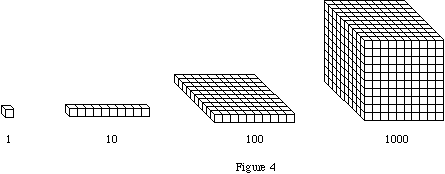The Lab Gear extends the model. In addition to yellow number blocks (ones, fives, and twenty-fives) there are "variable" blocks: x, y, xy, x2, xy, y2, x2y, xy2, x3, y3. [Figure 5]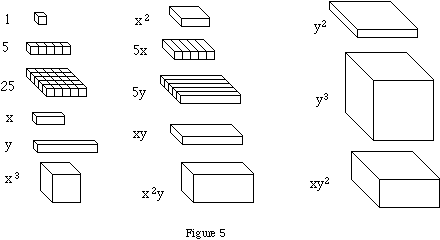### * Make a Rectangle

A canonical activity in this environment is:

a. Make a rectangle using a given set of blocks;

b. For this rectangle, write the multiplication 'length * width = area'

For example, making a rectangle with a certain number of units leads to the interesting discussion of how many such rectangles are possible. In the case where only one is possible, the number is prime.

Making a rectangle with three tens and six ones can only be done in the 3*12=36 arrangement. Doing a series of problems of this type can provide good preparation for a discussion of how to multiply one-digit numbers by two-digit numbers, and more generally for the distributive law.

Making a rectangle with three x's and six ones is in some ways the same problem, except that the multiplication is 3(x+2)=3x+6, a fairly explicit example of the distributive law. Again, doing a series of problems of this type can lay the groundwork for interesting discussions.

"Make a Rectangle" is a straightforward question that all students can understand. Actually finding the rectangle can be challenging, particularly in the case of a trinomial. (Say the required blocks are one x2, three x's, and two 1's.) Such a visual puzzle appeals to many students. Being able to "read" the multiplication in the resulting rectangle is helped by the use of the Lab Gear's Corner Piece, which helps students see the dimensions of the rectangle. The figure shows 3(2x+1)=6x+3 [Figure 6]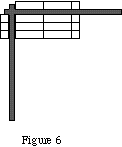Some understandings about operations are built into such a figure: that lining up two x's and 1 is a representation of x+x+1 or 2x+1, that the area of a rectangle is a representation of multiplication of its dimensions. These understandings cannot be taken for granted, and their discussion is part of the essential purpose of the activity, whose goal is only partially to factor the original expression. Developing visual models for addition and multiplication is pedagogically and mathematically very powerful. One cannot credibly argue that students are better off not understanding these models than understanding them.

On the other hand, I do not claim that merely doing these activities will accomplish miracles. To develop an understanding of the distributive law, and an ability to use it, takes a lot more. "Make a Rectangle" must be followed up with a range of activities, some using manipulatives, some not, and some making connections between the manipulatives and the other representations. "Make a Rectangle" starts with the area and asks for the dimensions. The activity can be reversed: given the sides, what is the area? This gives students a method for finding the product of 12 and 15, or of (x+2) and (y+x+1). After building the multiplications with blocks, students can sketch the block multiplication on paper, without blocks, and then they can be shown the "multiplication table" format for multiplying [Figure 7].

 5x +6 3x 15x2 +18x +4 +20x +24

Figure 7

These activities can be followed up or accompanied with work on division (given the area and one side of a rectangle, what is the other side?), with activities based on the volume of a rectangular prism ("Make a box, write an equation about its volume"), as well as with a study of patterns in squaring and cubing. Even after doing all this work, there is no guarantee that students will have mastered all the ideas that are involved. But the chances are much better than if the geometric representation was denied them. As a follow-up on this sort of work, in secondary school, algebra manipulatives can make such previously arcane topics as completing the square considerably more accessible.

### * Perimeter

Another activity altogether with the Lab Gear, which is also very relevant to the issue of understanding operations is the task: "find the perimeter of this Lab Gear figure" [Figure 8].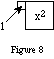This is a very useful activity for two reasons. On the one hand, students find one part of it quite difficult, which reveals their weak grasp of operations. (Specifically, the side of length x-1 often eludes them.) On the other hand, this is a rich arena for alternate strategies, and alternate solutions, which are interesting to discuss. For example, is the perimeter 4x+2 or 3+3x+(x-1)? How could both of these be right?

Some teachers object to this activity by bringing up that x and y cannot be variable in this context, since for example, negative values are meaningless. Indeed, this is a situation where x and y each stand for a given (unknown) value. This is a common way to use variables, and this activity provides a context where this subtlety can be discussed with students.

## Manipulatives Polemics

I cannot guarantee that the use of algebra manipulatives at the elementary level will be helpful. I have used other manipulatives at that level, and I have used algebra manipulatives at the secondary level, but since I developed my algebra manipulatives while working in a high school, I have no idea how they would be received by younger children. All I can say is that I think this would be an interesting and important area of research. My hunch, based on reactions of upper elementary school teachers, is that algebra manipulatives can be used profitably with students as young as 9 years of age. In any case, I would like to answer some of the general objections to manipulatives that I have heard from various math educators.

### * Dimensions

Some educators object to this use of manipulatives because it presumes an understanding of area (and volume) that not all students have. That is a legitimate concern, but all it means is that we need to learn how to help students develop those understandings. There are various ways to deal with that (see for example some ideas in the Smith and Thompson chapter in this volume). Not only do manipulatives in no way inhibit the learning of the concept of area -- in fact they provide both motivation and an additional arena for the teacher to pay attention to this crucial concept which has pay-offs and spin-offs all the way across the curriculum. If the fact that some students have a weak understanding of area meant we should avoid using area in teaching algebra, then we couldn't use numbers in teaching anything, since some students have a weak sense of number!

Beyond area and volume, some educators are concerned about the fact that in a Lab Gear problem like 3(2x+1)=6x+3, the x on the left side refers to the length of the block, while the x on the right side refers to its area [Figure 6]. Is this confusing to the students, they ask? Students do not usually know enough to be confused by this, but it is interesting for teachers to think about it.

The Lab Gear can serve as a model of dimensions with the following convention: a thickness of one unit does not contribute to the dimensionality of a block figure. From that point of view, x3 is a model of a three-dimensional object, xy is a model of a two-dimensional object, x is a model of a one-dimensional object, and 1 is a model of a zero-dimensional object. This is of course not literally true: all physical objects, including Lab Gear blocks, are three-dimensional. However this convention allows us to have a consistent model. The x on the left is in an (x+2) by 1 by 1 arrangement, so we think of it as one-dimensional, and hence x refers to its length. The x on the right is part of an (x+2) by 3 by 1 arrangement, so it is part of a two-dimensional figure, and x refers to area.

In this model, the degree of a polynomial is the lowest dimension that the blocks representing the polynomial can be arranged in. For example, three x-blocks can be arranged as a two-dimensional rectangle, or a one-dimensional line segment [Figure 9]. Since the lowest dimension is one, 3x is of degree 1.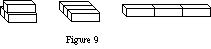All this may be too subtle for elementary school students, but it does not prevent them from using the manipulatives at an appropriate level. After all, we don't expect children to understand how calculators work, but that does not prevent the calculator from serving a purpose in their education! The learning of any new domain worth learning must necessarily rely on partial and incomplete understandings at some times.

### * Manipulatives as calculators

Yet others object to the use of manipulatives because they feel uncomfortable at the fact that manipulatives can make previously difficult work appear easy, and therefore can mask a lack of understanding. That is a crucial insight. Deborah Ball (1992) gives the example of students carrying out a subtraction correctly with manipulatives, by following rules they have memorized. However, once removed from the manipulatives, they revert to their previous mistakes. The very same thing happens with the example given above: students who can multiply binomials correctly with the help of the Lab Gear, will later write (x+5)2=x2+25. Does this mean that manipulatives should not be used? Absolutely not.

First of all, manipulatives obviously do not cause the mistake, which happened before manipulatives even existed. Second, if a student can do this multiplication correctly with manipulatives, upon making this mistake when working with the symbols, mere mention of the manipulatives by the teacher is often enough for the student to think about it, perhaps draw a sketch, and make the correction without any further guidance. In other words manipulatives can provide a student-controlled home base to which they can retreat as necessary, until they no longer need it. Obviously, the goal is to get rid of the training wheels, to use Ball's metaphor, or the scaffolding, in Mason's words, but that is not necessarily quick or easy. Mathematical understanding can only be acquired through arduous struggle. There is no royal road.

Moreover, the use of a tool to "get the answer" is not wrong in principle. There are times when it is appropriate for students to use technology (whether a calculator, a symbol manipulator, or manipulatives) to get the answer to a subtraction, or to square a binomial. The focus of a particular lesson, perhaps a "real world" application that involves subtraction, may not be on the mechanics of subtracting correctly, or on place value. In such a situation, students need to get the right answer quickly, whether or not their conceptual understanding of how it is computed is solid.

If we were to deny students access to tools that make this possible, we would be joining forces with those who are trying to prevent students from using calculators, and would have little chance of getting very far. By the way, another way to think about the "problem" that manipulatives make difficult things easy is that they help weaker students pass required classes and exams -- a short-term but real issue for them. They do not have the luxury we have to say that those classes and exams --which most of us passed comfortably and thereby gained access to the academy-- are garbage. As one of my students put it: "The Lab Gear saved my butt!"

Of course, there should be times when the focus of the lesson is on place value and on comparing strategies for subtracting two-digit numbers. At such times, we may use manipulatives, or not, but in either case, we need to devise lessons which will get to the underlying concepts, not merely on "getting the answer." This is best achieved through discussion.

### * Conversations

Some educators object to the use of manipulatives because they do not reflect mathematical abstractions accurately enough. For example, it is pretty much impossible to represent certain fractions with base ten blocks, and the Lab Gear blocks that represent variables are of a fixed size, which seems to be a contradiction in terms.

However it is not the primary role of a transitional environment for learning to be accurate. At the limit, the most accurate representation we have of the concepts of algebra is the traditional notation. If accuracy of model is all that is needed for pedagogical effectiveness, our job would be easy! In fact, the primary purpose of manipulatives is not to be accurate, but to offer an opportunity for discussion among students, and for discussion between the different cultures of student and teacher. Jeremy Roschelle (1996) conducts an outstanding discussion of this issue in the context of designing educational software for teaching physics. He correctly concludes: "... rather than merely representing mental models accurately, designers must focus on supporting communicative practices." In fact it turns out that the limitations of the manipulative model provides the spark for some of the best discussions in the classroom.

Here is an anecdote related by Pat Thompson:

A student suggested that the "thousand" base-10 block could be replaced by an apple. The teacher asked what would then be used to represent 100, 10, and 1. The student replied that apples could be used for everything.

This is a great anecdote, which shows the power of manipulatives as a conversation starter. The student may have made the suggestion because he was grappling with the question "how could this one block represent 1000?" -- a profound question. Or perhaps the student has no clue about how 1000 differs from 100, or 10 or 1. In any case, the student would not have been better off if the base ten blocks had been excluded from the classroom. It is precisely because of their presence that an opportunity for a discussion of how and why one block can represent 1000 (or how and why 1000 is different from 100, etc) arose. In most classrooms students do not have the opportunity or the context to verbalize their thoughts and misunderstandings. A single discussion is not sufficient, of course, but a fruitful discussion of student-designed representations for our numeration system could follow the student's suggestion about apples, using apples, buttons or whatever. After such a discussion over a period of days, the teacher (with the help of those students who already understand it) may be able to re-introduce the base 10 blocks in a way that would be more meaningful to the student. Clearly, while understanding the model cannot be equated with understanding the concept, not understanding base ten blocks is diagnostic of not understanding numeration in base ten.

To take another example, consider the story of Adam, the fourth grader who found that "if you take two consecutive numbers, you add the lower number and its square to the higher number, and you get the higher number's square" (Bastable and Schifter, in this volume). As Bastable points out, writing this in algebraic notation is enough to prove the correctness of Adam's assertion. However, in fourth grade this route is closed, since to a nine-year old this amounts to meaningless gibberish. A priori, the only way at that level to check the validity of that assertion is to try it with various numbers, which is indeed what was done. But another way is open if we keep in mind the strategy used by the students in Rigoletti's third grade, who represented square numbers with square arrangements of unit squares. [figure 10] An extra level in the process of generalization could be seen in the Lab Gear representation of the same phenomenon.Such an arrangement could be arrived at through questions such as the following [figure 11]: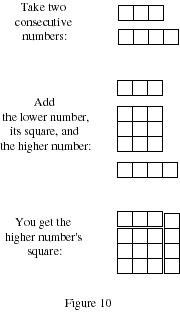How would you represent two consecutive numbers with the Lab Gear?
How would you represent the square of each of these numbers?
How would you represent Adam's conjecture?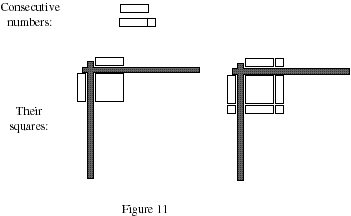A highly skilled teacher is able to generate worthwhile discussions with students with or without manipulatives. Manipulatives, because they are, in Papert's (1980) terminology "objects to think with" and objects to talk about, have the potential to improve discourse in more classes. They are often a teacher's first step away from traditional instruction, and they can lead to decisive changes in classroom culture: the introduction of collaborative work, the option for students to create exercises instead of merely solving them, an opportunity for the teachers to enjoy a new window on student understanding or lack of it, and so on.

### * States vs actions

Jim Kaput (in press) argues that computer-based "manipulatives" would have advantages over physical ones, because of the computer's ability to record processes, or to display variables with varying sizes. It may well be that such software could be designed, tested, and distributed to schools along with the necessary hardware. However, the reality is that physical manipulatives will reach many, many more classrooms much, much sooner. So it is useful to ponder whether what Kaput calls the "eternal present" of manipulatives can be transcended with adequate design, lessons, and teaching.

Kaput points out that when modeling 2+3=5 with unifix cubes, for example, by the time one has combined 2 and 3 blocks together, one sees 5 blocks, but the original 2 and 3 blocks, as well as the process of combining them has vanished. True enough, though he admits that color can help overcome this. For multiplication, the Lab Gear corner piece allows students to see both the factors and the products when multiplying, or factoring. This is a design solution. But more significantly, the activity "add 2 to 3 using Unifix cubes" is just about the least interesting activity imaginable in this domain. Using Krutetskii's and Rachlin's insights, we can readily come up with the following improvement: "What are all the ways that you can combine sets of Unifix cubes in order to get a total of 5 cubes?" This encourages students to keep records of which pairs worked, gives them a chance to notice patterns (using one less red cube requires using one more green cube, for example), and focuses their attention on the process rather than (or in addition to) the result. It also provides the springboard to a question for the top students in the class, who can start investigating the question of how many partitions of 5 are possible.

Kaput also argues that the computer makes it possible to have "hot" links between representations on the screen. In other words, when you manipulate symbols on the screen, images of blocks could react to the manipulations as you go. This is nice, and some software along these lines exists to show the connections between different representations of functions. However, we should not be lulled into thinking that an on-screen connection guarantees a connection in the student's mind. The key question is not one of equipment -- it is how to use the available equipment well. In spite of Papert's predictions (1980), the technology of the last 15 years did not bring about an educational revolution. I see no reason to believe this will change in the next 15 years.

## Tool-based Learning

Deborah Ball, in a brilliant paper (1992), warns against the "magical hopes" that many teachers have about manipulatives. She writes: "Manipulatives -- and the underlying notion that understanding comes through the finger tips -- have become part of educational dogma." She gives several examples of the mere use of manipulatives failing to deliver understanding, and concludes that manipulatives cannot be used effectively without adequate teacher preparation, and without better understanding of how children learn. She also acknowledges that "Manipulatives undoubtedly have a role to play ... by enhancing the modes of learning and communication available to our students." I strongly agree with Ball's concerns and insights, and do not claim magical powers for manipulatives. In fact, in my own teaching, manipulatives have always been one tool among many. I use computers and calculators, graph paper, and group discussion. Sketches on the chalkboard, whether drawn by me or by my students, play a crucial role in my class, and probably in any effective math teachers' class. The use of any one tool is not a rejection of others!

On the other hand, teachers' interest in manipulatives provides us with an opportunity to work with them. In the past few years, I have been asked to introduce in-service and pre-service teachers to the Lab Gear dozens of times. These workshops have given me the opportunity to work with hundreds, perhaps thousands of teachers, and the discussion inevitably goes beyond the technicalities of the Lab Gear, to important mathematical, curricular, and pedagogical questions, such as the shift in emphasis from "skills" to conceptual understanding.

At any rate, over years of work with both students and teachers, I have learned that:

• Manipulatives are an extraordinary tool to help reach weaker students, but that is not their only purpose: they are a useful way to improve education in any math class.
• Often, teachers are ineffective because of their own limited understanding of the material. Manipulatives provide an environment to teach math as well as pedagogy to teachers.
• Manipulatives do not make math "easy", and teachers may need to learn something in order to use them. The increased understanding will serve them whether or not they use manipulatives in their class in the immediate future.
• There is no sense in using manipulatives in a "do as I say" algorithmic model which only perpetuates antiquated pedagogy. It is far more effective to use them as a setting for problem solving, discussion, communication, and reflection.
• Manipulatives should be a complement to, not a substitute for other representations. In particular, Cartesian graphing and other pictorial representations are extremely important.
• Deliberate attention must be paid to help students transfer what they know in the context of the manipulatives to other representations, including symbolic, numerical, and graphical. Transfer does not just happen spontaneously.

The purpose of manipulatives is to enhance discourse, and to provide a locus for the more theoretical discussion of the numeration system (in the case of base 10 blocks) or of the structures underlying aspects of the field structure of the number system and its algebra (in the case of both Base 10 blocks and the Lab Gear). That theoretical dimension runs the risk of being buried or forgotten if we go full-steam into the currently fashionable direction of "real world" problems.

## Fun Math

In most schools, early mathematics means arithmetic. Many elementary school teachers are not comfortable with math topics beyond basic arithmetic. Most people, including elementary school students and their parents, are convinced that mathematics is arithmetic. Until recently, the NCTM journal for elementary school teachers was called The Arithmetic Teacher, revealing that confusion even among the leaders in the profession.

Below, I list some "fun" domains for early mathematics. For each domain, I hint at some interesting problems or materials, most of which I have used with young children in my ten years as an elementary school math specialist and classroom teacher. Obviously, this is not a comprehensive curriculum, just an attempt at giving some substance to the claim that a lot more than arithmetic is possible in elementary school.

### * Perimeter Patterns

Pattern blocks [Figure 12] are available in many elementary schools. They can be used as a source of interesting perimeter pattern problems.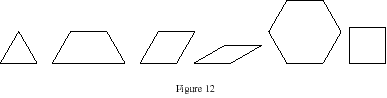For example, by making a figure with alternating squares and hexagons, [Figure 13], we get the following sequence of perimeters: 4, 8, 10, 14, 16, 20, 22, ... The sequence is rich in patterns that students can explore. What kinds of numbers can be found on this list? What kinds of numbers cannot? What would the hundredth number be?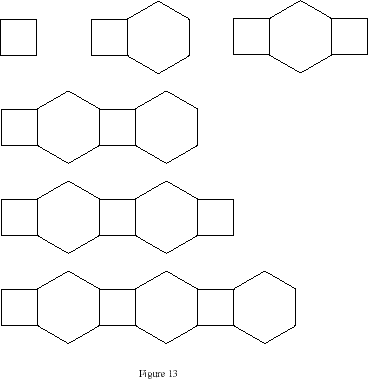A simpler sequence is obtained by adding the square and hexagon as a unit, yielding the sequence: 8, 14, 20, 26, ... Simpler yet are sequences built out of using only squares or only hexagons. Such sequences are arithmetic, and students can learn to represent them symbolically.

Another question from the same domain: Is it possible to create a pattern block figure with an odd perimeter?

### * Angles

The same blocks can be used to introduce the concept of angle, which is notoriously difficult to introduce to tenth graders if they haven't worked with it before. Here is a sequence of activities using the pattern blocks. (Note: pattern block hexagons are yellow, squares are orange, and so on.) These will take several days to complete.

1. In how many ways can you surround a point with pattern blocks using only yellow blocks? using only blue blocks? using two colors? etc.
2. There are 360 degrees around a point. Use this information to find the measurement of the angles in each pattern block.
3. What is the sum of the angles in each of the pattern blocks? Using one or more pattern blocks, make a polygon for which the sum of the angles is greater than that for the quadrilaterals, but less than that for the hexagon. Create a 7-gon, an 8-gon, etc with pattern blocks. In each case, find the sum of the angles. What is the pattern?
4. Polygon walks: give instructions to a classmate to walk each pattern block shape. (Example: take a step forward, turn right 90 degrees, etc.) Give instructions to walk a two-pattern block polygon. This activity can lead to turtle geometry work on computers.

### * Geometric Puzzles:

Geometric puzzles such as tangrams and pentominoes offer opportunities for lessons on similarity, congruence, symmetry, area, and more. (Picciotto, 1986. See also EDC's Elementary Science Study _Tangrams_ unit from the sixties.)

I will give two examples from the world of pentominoes. Pentominoes are the twelve distinct shapes that can be obtained by joining five squares edge-to-edge [figure 14].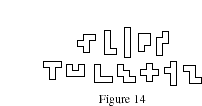1. What rectangles can be covered exactly by one or more pentominoes from one set ? (Pentominoes cannot be overlapped or reused.) To get this discussion started, students can try to cover rectangles such as: 3 by 5, 2 by 5, 2.5 by 6, 2 by 10, 3 by 6, and so on. Students discover that pentomino rectangles must satisfy these conditions:
• whole number sides
• an area that is a multiple of 5, between 5 and 60, inclusive (except for area 10, surprisingly)
2. If you double (or triple) the dimensions of a given pentomino, can the resulting shape be covered with pentominoes? How many pentominoes are needed to do it?

Many other interesting questions can be asked about pentominoes, and about the generalized polyominoes. A related domain is superTangrams, shapes obtained by joining four isosceles right triangles edge-to-edge. In this case, problem 2 above becomes a lot richer and more complex, because in addition to doubling and tripling the dimensions, it becomes possible to get similar figures with area equal to twice or eight times the original area.

### * Lattice geometry:

Geoboards and dot paper offer opportunities for lessons on area (including Pick's formula), Cartesian coordinates, ratio, similarity, slope, and more.

Two examples for upper elementary school:

1. How many different-sized squares can one find in an 11 by 11 geoboard? This leads to a major hunt which includes experiences about slope (how to find perpendicular sides if the vertices are all on lattice points), and area (the area of a "tilted" square can be obtained without the Pythagorean Theorem by subtracting right triangles from "horizontal" squares). (See Picciotto and Wah, 1993 for more on this.)
2. Pick's formula relates the area of a polygonal shape with all vertices on lattice points to the number of lattice points on the circumference, and to the number of interior lattice points. With some guidance and much exploration, students can discover this formula.

Many interesting explorations can also happen at a more elementary level, and others (leading to strengthen ideas about angles) are possible on the so-called circular geoboard, which features pegs spaced at 15 degree intervals on a circle, as well as a central peg.

### * Graph Theory:

The Koenigsberg bridge problem, Euler's formula on the plane or in polyhedra. The two-color theorem (what maps can be colored in two colors? how can one change a three- or four-color map to a two color map by adding edges?)

The discovery of Euler's formula, like Pick's, makes for particularly interesting lessons, because they each involve three variables instead of the usual two -- which makes for more protracted investigations.

And there are more arenas for the development of early mathematical thinking: programmable computer environments such as Logo, Boxer, spreadsheets, etc; logic with attribute blocks, the game of Set, etc.; art with tiling, symmetry, Escher and Islamic designs; data analysis and probability; etc. I hope it is clear that children need not be fed only a steady diet of number crunching. Plenty of interesting mathematical work can be done in a wide range of mathematical domains such as the ones listed above.

While I in no way want to diminish the importance of work with numbers, we must acknowledge that by de-emphasizing multi-digit paper-pencil arithmetical algorithms at the upper elementary levels, we are opening up hundreds of hours for other mathematical work. Instead of filling that with an attempt to teach the traditional Algebra 1 course to younger and younger students (an unfortunate trend in many school districts), we should seize the opportunity to broaden the curriculum. The point is not that students need to know Euler's formula in sixth grade, but that they need to be engaged in worthwhile mathematical thinking at an appropriate level.

## Conclusion

I have tried to articulate a vision of elementary school mathematics that would be considerably richer than the current fixation on arithmetic. It is also richer than some reform proposals. The following maps may help us rethink early mathematics:

Where we are:

 Arithmetic ---> "Algebra 1" ---> Secondary Math

Where we should go (with an emphasis on operations throughout):

 Arithmetic and number theory Quantitative reasoning and the rational numbers "Fun Math" as described above Manipulatives and computer programming (to support the above) ---> Secondary Math

That vision stems from some political assumptions, and it has political consequences.

The assumptions: mathematics education is not just about preparing students for "practical" matters and helping the economy. It is an important part of human culture of sense-making, and should be introduced as such to all students from a young age. In addition to being useful, math is fun and beautiful. We should not lose sight of this as we attempt to make the curriculum more relevant through greater reliance on applications.

The consequences: we need math specialists in all elementary schools. It is not likely that this vision or anything like it can be implemented without having people with at least a BA in mathematics as well as some pedagogical sophistication involved in the front lines. This is more important than any particular curricular decision, because such people in the schools would transform the daily experience of math education with whatever curriculum.

One could argue that a massive effort aimed at training elementary school teachers, through both pre-service and in-service work, is needed. I don't disagree with this, but one math specialist can work with approximately ten classrooms, and provide an on-going presence that will have much more impact on teachers than a one-shot training session. That ten-to-one ratio also means that teacher-training budgets can have an impact that is one order of magnitude greater.

Finally, a national campaign to put math specialists in all elementary schools is a program that teachers and parents in all communities could support even if they are not ready to make major curricular changes. Who knows, they may be able to talk politicians, funding agencies, and corporations into supporting such an effort. Whether or not we succeed in making curricular changes in the short run, a national corps of math specialists could be the communication line (in all directions) between the mathematics community, the math ed community, and the elementary schools' students, parents, and teachers.

## Bibliography

Abraham Arcavi, "Symbol Sense: informal sense-making in formal mathematics" For the Learning of Mathematics, November 1994, vol 14(3), pp. 24-35.

Deborah Ball, "Magical Hopes: Manipulatives and the Reform of Math Education", American Educator, Summer 1992

College Entrance Examination Board, Changing the Odds New York: CEEB 1990

James Kaput, "Overcoming Physicality and the Eternal Present: Cybernetic Manipulatives", in R. Sutherland and J. Mason (Eds), Visualization and technology in mathematics education, New York, NY: Springer (in press)

Seymour Papert, Mindstorms, 1980

Henri Picciotto, Pentomino Lessons, Mountain View, CA: Creative Publications, 1986

Henri Picciotto, Polyomino Lessons, Mountain View, CA: Creative Publications, 1986 (out of print)

Henri Picciotto and Anita Wah, "A new Algebra: Tools, Themes, Concepts" (Journal of Mathematical Behavior, volume 12, #1, March 1993)

Sidney L. Rachlin. "Algebra from x to why: A Process Approach for Developing the Concepts and Generalizations of Algebra." In Wendy Caughey (Ed.), From Now to the Future. Melbourne, Australia: The Mathematical Association of Victoria, 1987, 213-217.

Jeremy Roschelle (1996). Designing for cognitive communication: epistemic fidelity or mediating collaborating inquiry. In D.L. Day and D.K. Kovacs (Eds). Computers, Communication and Mental Models. London: Taylor and Francis. 13-25.

Anita Wah and Henri Picciotto, Algebra: Themes, Tools, Concepts, Mountain View, CA: Creative Publications, 1994.

I would like to thank Jim Kaput and Abraham Arcavi for their comments on a previous version of this paper.Related pages on this site:
Middle School
Fractions on Grid Paper
Slices
The Egyptian Fractions Challenge
Tool-Based Pedagogy
A New Algebra
Lab Gear
Geometric Puzzles in the Classroom
Pattern Blocks

Elsewhere:
Working with Pentominoes by Henri Picciotto